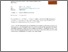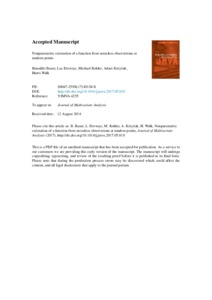Title:

# Nonparametric estimation of a function from noiseless observations at random points

Bauer, Benedikt, Devroye, Luc, Kohler, Michael, Krzyżak, Adam and Walk, Harro (2017) Nonparametric estimation of a function from noiseless observations at random points. Journal of Multivariate Analysis . ISSN 0047259X (In Press)Preview
Text (application/pdf)
Nonparametric-estimation-of-a-function-from-noiseless-observations-at-random-points_2017_Journal-of-Multivariate-Analysis.pdf - Accepted Version
1MB

Official URL: http://dx.doi.org/10.1016/j.jmva.2017.05.010

## Abstract

In this paper we study the problem of estimating a function from n noiseless observations of function values at randomly chosen points. These points are independent copies of a random variable whose density is bounded away from zero on the unit cube and vanishes outside. The function to be estimated is assumed to be (p,C)-smooth, i.e., (roughly speaking) it is p times continuously differentiable. Our main results are that the supremum norm error of a suitably defined spline estimate is bounded in probability by {ln(n)∕n}p∕d for arbitrary p and d and that this rate of convergence is optimal in minimax sense.

Divisions: Concordia University > Gina Cody School of Engineering and Computer Science > Computer Science and Software Engineering Article Yes Bauer, Benedikt and Devroye, Luc and Kohler, Michael and Krzyżak, Adam and Walk, Harro Journal of Multivariate Analysis 19 June 2017 Natural Sciences and Engineering Research Council of Canada 10.1016/j.jmva.2017.05.010 Multivariate scattered data approximation; Rate of convergence; Supremum norm error 982656 DANIELLE DENNIE 03 Jul 2017 12:44 01 Jun 2018 00:00

## References:

J. Beirlant, L. Györfi On the asymptotic L2-error in partitioning regression estimation J. Statist. Plann. Inference, 71 (1998), pp. 93–107

L. Devroye Necessary and sufficient conditions for the almost everywhere convergence of nearest neighbor regression function estimates Z. Wahrscheinlichkeitstheor. Verwandte Geb., 61 (1982), pp. 467–481

L. Devroye, L. Györfi, A. Krzyzak, G. Lugosi On the strong universal consistency of nearest neighbor regression function estimates Ann. Statist., 22 (1994), pp. 1371–1385

L. Devroye, A. Krzyżak An equivalence theorem for L1 convergence of the kernel regression estimate J. Statist. Plann. Inference, 23 (1989), pp. 71–82

L. Devroye, T. Wagner Distribution-free consistency results in nonparametric discrimination and regression function estimation Ann. Statist., 8 (1980), pp. 231–239

R. Farwig Multivariate interpolation of arbitrarily spaced data by moving least squares methods J. Comput. Appl. Math., 16 (1986), pp. 79–93

L. Györfi Recent results on nonparametric regression estimate and multiple classification Prob. Control Inf. Theory, 10 (1981), pp. 43–52

L. Györfi, M. Kohler, A. Krzyzak, H. Walk A Distribution-Free Theory of Nonparametric Regression, Springer, New York (2002)

M. Johnson, Z. Shen, Y. Xu Scattered data reconstruction by regularization in b-spline and associated wavelet spaces J. Approx. Theory, 159 (2009), pp. 197–223

G. Joldes, H. Chowdhury, A. Wittek, B. Doyle, K. Miller Modified moving least squares with polynomial bases for scattered data approximation Appl. Math. Comput., 266 (2015), pp. 893–902

M. Kohler Inequalities for uniform deviations of averages from expectations with application to nonparametric regression J. Statist. Plann. Inference, 89 (2000), pp. 1–23

M. Kohler Optimal global rates of convergence for noiseless regression estimation problems with adaptively chosen design J. Multivariate Anal., 132 (2014), pp. 197–208

M. Kohler, A. Krzyżak Nonparametric regression estimation using penalized least squares IEEE Trans. Inform. Theory, 47 (2001), pp. 3054–3058

M. Kohler, A. Krzyżak Optimal global rates of convergence for interpolation problems with random design Statist. Probab. Lett., 83 (2013), pp. 1871–1879

P. Lancaster, K. Salkauskas Surfaces generated by moving least squares methods Math. Comp., 37 (1981), pp. 141–158

D. Lazzaro, L. Montefusco Radial basis functions for the multivariate interpolation of large scattered data sets J. Comput. Appl. Math., 140 (2002), pp. 521–536

Q. Le Gia, F. Narcowich, J. Ward, H. Wendland Continuous and discrete least-squares approximation by radial basis functions on spheres J. Approx. Theory, 143 (2006), pp. 124–133

D. Levin The approximation power of moving least-squares Math. Comp., 67 (1998), pp. 1517–1531

G. Lugosi, K. Zeger Nonparametric estimation via empirical risk minimization IEEE Trans. Inform. Theory, 41 (1995), pp. 677–687

W. Madych, S. Nelson Bounds on multivariate polynomials and exponential error estimates for multiquadric interpolation J. Approx. Theory, 70 (1992), pp. 94–114

C. Micchelli Interpolation of scattered data: distance matrices and conditionally positive definite functions Constr. Approx., 2 (1986), pp. 11–22

E. Nadaraya On estimating regression Th. Probab. Appl., 9 (1964), pp. 141–142

E. Nadaraya Remarks on nonparametric estimates for density functions and regression curves Th. Probab. Appl., 15 (1970), pp. 134–137

F. Narcowich, J. Ward, H. Wendland Sobolev error estimates and a bernstein inequality for scattered data interpolation via radial basis functions Constr. Approx., 24 (2006), pp. 175–186

Y. Ohtake, A. Belyaev, H. Seidel 3D scattered data interpolation and approximation with multilevel compactly supported RBFs Graph. Models, 67 (2005), pp. 150–165

Y. Ohtake, A. Belyaev, H. Seidel Sparse surface reconstruction with adaptive partition of unity and radial basis functions Graph. Models, 68 (2006), pp. 15–24

T. Sauer Polynomial interpolation in several variables: lattices, differences, and ideals in: K. Jetter, M. Buhmann, W. Haussmann, R. Schaback, J. Stöckler (Eds.), Topics in Multivar. Approx. and Interpolation, Stud. Comput. Math., 12, (2006), pp. 191–230

C. Stone Consistent nonparametric regression Ann. Statist., 5 (1977), pp. 595–645

C. Stone Optimal global rates of convergence for nonparametric regression Ann. Statist., 10 (1982), pp. 1040–1053

G. Wahba Spline Models for Observational Data, SIAM, Philadelphia, PA (1990)

G. Watson Smooth regression analysis Sankhyā Ser. A, 26 (1964), pp. 359–372

H. Wendland Local polynomial reproduction and moving least squares approximation IMA J. Numer. Anal., 21 (2001), pp. 285–300

H. Wendland Scattered Data Approximation, Cambridge University Press, Cambridge, UK (2005)
All items in Spectrum are protected by copyright, with all rights reserved. The use of items is governed by Spectrum's terms of access.

Repository Staff Only: item control pageResearch related to the current document (at the CORE website)
Back to top